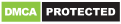# Angular 10 Decimal Pipe: Format Numbers by Example

In this example, we'll learn how to use the Angular Decimal Pipe to format numbers.

You'll need to have a few prerequisites for this tutorial such as Node.js and Angular CLI v10 installed on your local development machine.

## Angular 10 Decimal Pipe by Example

Next, you'll need to create a project using the following command:

``````\$ ng new Angular10DecimalPipeExample
``````

The CLI will ask you a couple of questions — If Would you like to add Angular routing? Type y for Yes and Which stylesheet format would you like to use? Choose CSS.

Angular Decimal Pipe is a builin pipe in Angular that can be used to format decimal numbers.

## How to Use Angular Decimal Pipe

Angular decimal pipe accepts two parameters, the decimal digit info and locale:

``````
``````

Open the `src/app/app.component.ts` file and add a variable of type number as follows:

``````export class App implements OnInit {

aNumber: number = 10.123456789;

constructor() { }
ngOnInit() {
}

}
``````

Next, open the `src/app/app.component.html` file and update it as follows to use the decimal pipe without parameters:

``````
``````

The result will be:

// 10.123

Now change the information given to the decimal pipe as follows:

``````
``````

The result will be:

// 0010.123456

Since our number contains only two digits before decimal point two extra zeroes are padded.

In our example we configure the decimal pipe to display at least 4 digits before the decimal point, minimum 1 digit, and maximum 6 digits after the decimal point.

## Angular Decimal Pipe with Country Locale

You can also format numbers in your templates according to a country locale. You need to pass the country locale code as a second parameter to the decimal pipe. For example:

``````
``````

## How to Use the Decimal Pipe in Components in Angular 10

You can also use the decimal pipe from your component's class. Let's see an example.

First, open the `src/app/app.module.ts` file and update it as follows:

``````import { NgModule,  } from '@angular/core';
import {DecimalPipe} from '@angular/common';
import { BrowserModule } from '@angular/platform-browser';
import { FormsModule } from '@angular/forms';

import { AppComponent, CustomFormatterPipe } from './app.component';

@NgModule({
imports:      [ BrowserModule ],
declarations: [ AppComponent],
providers: [DecimalPipe],
bootstrap:    [ AppComponent,  ]
})
export class AppModule { }
``````

We import `DecimalPipe` from `@angular/common` and add it to the `providers` array of the module.

Next, open the `src/app/app.component.ts` file and update it as follows:

``````import { Component } from '@angular/core';
import {DecimalPipe} from '@angular/common';

@Component({
selector: 'my-app',
template:`
<div> </div>
`,
styleUrls: [ './app.component.css' ]
})
export class AppComponent  {
num = 1.3765273;
format = '2.0-2';
result = null;

constructor(private decimalPipe: DecimalPipe) { }

ngOnInit(){

this.result = this.decimalPipe.transform(this.num, this.format);
}

}
``````

We first import `DecimalPipe` from `@angular/core`. Next, we inject it via the component's constructor.

We define three variables `num`, `format` and `result`. Finally we call the `transform()` method of `DecimalPipe` -- we pass in the `num` variable as the first parameter and the `format` as the second parameter. The formatted number will be returned and assigned to the `result` variable.

## Conclusion

In this example, we've seen how to use decimal pipe in our Angular 10 app both in templates and component's classes.